# Forces- IntroductionPage 1

#### WATCH ALL SLIDES

Slide 1FORCES

Slide 2## What is a force?

A force is an influence on a system or object which, acting alone, will cause the motion of the system or object to change. If a system or object at rest is subjected to a non-zero force it will start to move.

Slide 3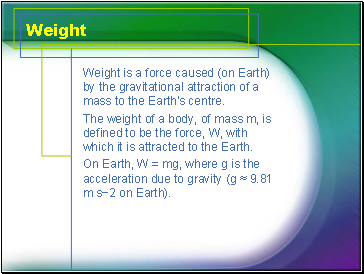## Weight

Weight is a force caused (on Earth) by the gravitational attraction of a mass to the Earth’s centre.

The weight of a body, of mass m, is defined to be the force, W, with which it is attracted to the Earth.

On Earth, W = mg, where g is the acceleration due to gravity (g ≈ 9.81 m s−2 on Earth).

Slide 4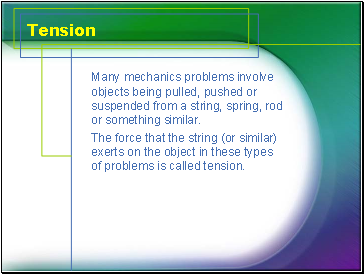## Tension

Many mechanics problems involve objects being pulled, pushed or suspended from a string, spring, rod or something similar.

The force that the string (or similar) exerts on the object in these types of problems is called tension.

Slide 5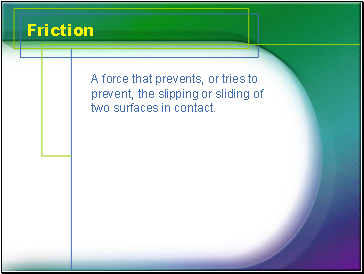## Friction

A force that prevents, or tries to prevent, the slipping or sliding of two surfaces in contact.

Slide 6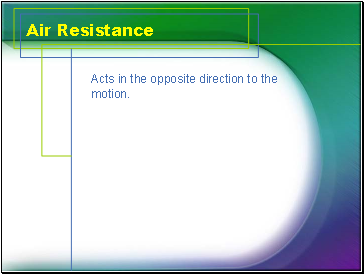## Air Resistance

Acts in the opposite direction to the motion.

Slide 7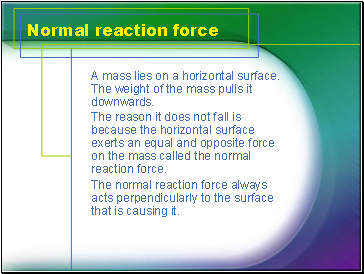## Normal reaction force

A mass lies on a horizontal surface. The weight of the mass pulls it downwards.

The reason it does not fall is because the horizontal surface exerts an equal and opposite force on the mass called the normal reaction force.

The normal reaction force always acts perpendicularly to the surface that is causing it.

Slide 8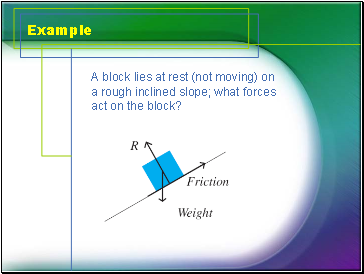## Example

A block lies at rest (not moving) on a rough inclined slope; what forces act on the block?

Slide 9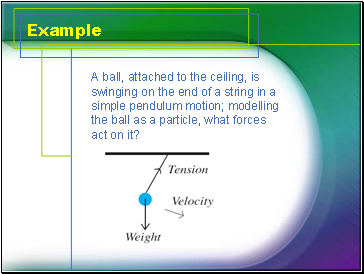Example

A ball, attached to the ceiling, is swinging on the end of a string in a simple pendulum motion; modelling the ball as a particle, what forces act on it?

Slide 10Example

What forces act on a trailer attached to a tow rope being pulled by a car?